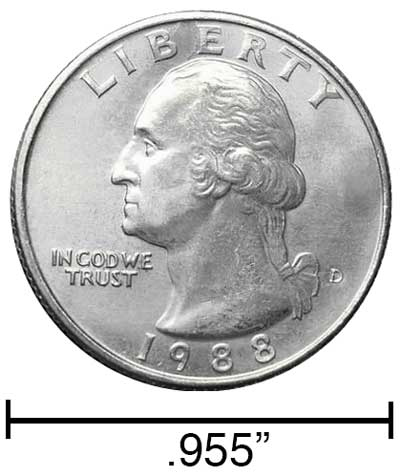# Miles to Inches Conversion

Enter the length in miles below to get the value converted to inches.

Results in Inches:1 mi = 63,360 in
Do you want to convert inches to miles?

## How to Convert Miles to InchesTo convert a mile measurement to an inch measurement, multiply the length by the conversion ratio.

Since one mile is equal to 63,360 inches, you can use this simple formula to convert:

inches = miles × 63,360

The length in inches is equal to the miles multiplied by 63,360.

For example, here's how to convert 5 miles to inches using the formula above.
5 mi = (5 × 63,360) = 316,800"

### How Many Inches are in a Mile?

There are 63,360 inches in a mile, which is why we use this value in the formula above.

1 mi = 63,360"

Our inch fraction calculator can add miles and inches together, and it also automatically converts the results to US customary, imperial, and SI metric values.

## Miles

The mile is a linear measurement of length equal to exactly 1,609.344 meters. One mile is also equal to 5,280 feet or 1,760 yards.

The mile is a US customary and imperial unit of length. Miles can be abbreviated as mi, and are also sometimes abbreviated as m. For example, 1 mile can be written as 1 mi or 1 m.

## Inches

An inch is a unit of linear length measure equal to 1/12 of a foot or 1/36 of a yard. Because the international yard is legally defined to be equal to exactly 0.9144 meters, one inch is equal to 2.54 centimeters.

The inch is a US customary and imperial unit of length. Inches can be abbreviated as in; for example, 1 inch can be written as 1 in.

Inches can also be denoted using the symbol, otherwise known as a double-prime. Often a double-quote (") is used instead of a double-prime for convenience. A double-prime is commonly used to express 1 in as 1″.The standard ruler has 12", and is a common measuring tool for measuring inches. They are also often measured using tape measures, which commonly come in lengths from 6' - 35'. Other types of measuring devices include scales, calipers, measuring wheels, micrometers, yardsticks, and even lasers.

We recommend using a ruler or tape measure for measuring length, which can be found at a local retailer or home center. Rulers are available in imperial, metric, or combination with both values, so make sure you get the correct type for your needs.

Need a ruler? Try our free downloadable and printable rulers, which include both imperial and metric measurements.

## Mile to Inch Conversion Table

Mile measurements converted to inches
Miles Inches
0.0001 mi 6.336"
0.0002 mi 12.67"
0.0003 mi 19.01"
0.0004 mi 25.34"
0.0005 mi 31.68"
0.0006 mi 38.02"
0.0007 mi 44.35"
0.0008 mi 50.69"
0.0009 mi 57.02"
0.001 mi 63.36"
0.002 mi 126.72"
0.003 mi 190.08"
0.004 mi 253.44"
0.005 mi 316.8"
0.006 mi 380.16"
0.007 mi 443.52"
0.008 mi 506.88"
0.009 mi 570.24"
0.01 mi 633.6"
0.02 mi 1,267"
0.03 mi 1,901"
0.04 mi 2,534"
0.05 mi 3,168"
0.06 mi 3,802"
0.07 mi 4,435"
0.08 mi 5,069"
0.09 mi 5,702"
0.1 mi 6,336"
0.2 mi 12,672"
0.3 mi 19,008"
0.4 mi 25,344"
0.5 mi 31,680"
0.6 mi 38,016"
0.7 mi 44,352"
0.8 mi 50,688"
0.9 mi 57,024"
1 mi 63,360"

## References

1. National Institute of Standards and Technology, Checking the Net Contents of Packaged Goods, Handbook 133 - 2019 Edition, https://nvlpubs.nist.gov/nistpubs/hb/2019/NIST.HB.133-2019.pdf